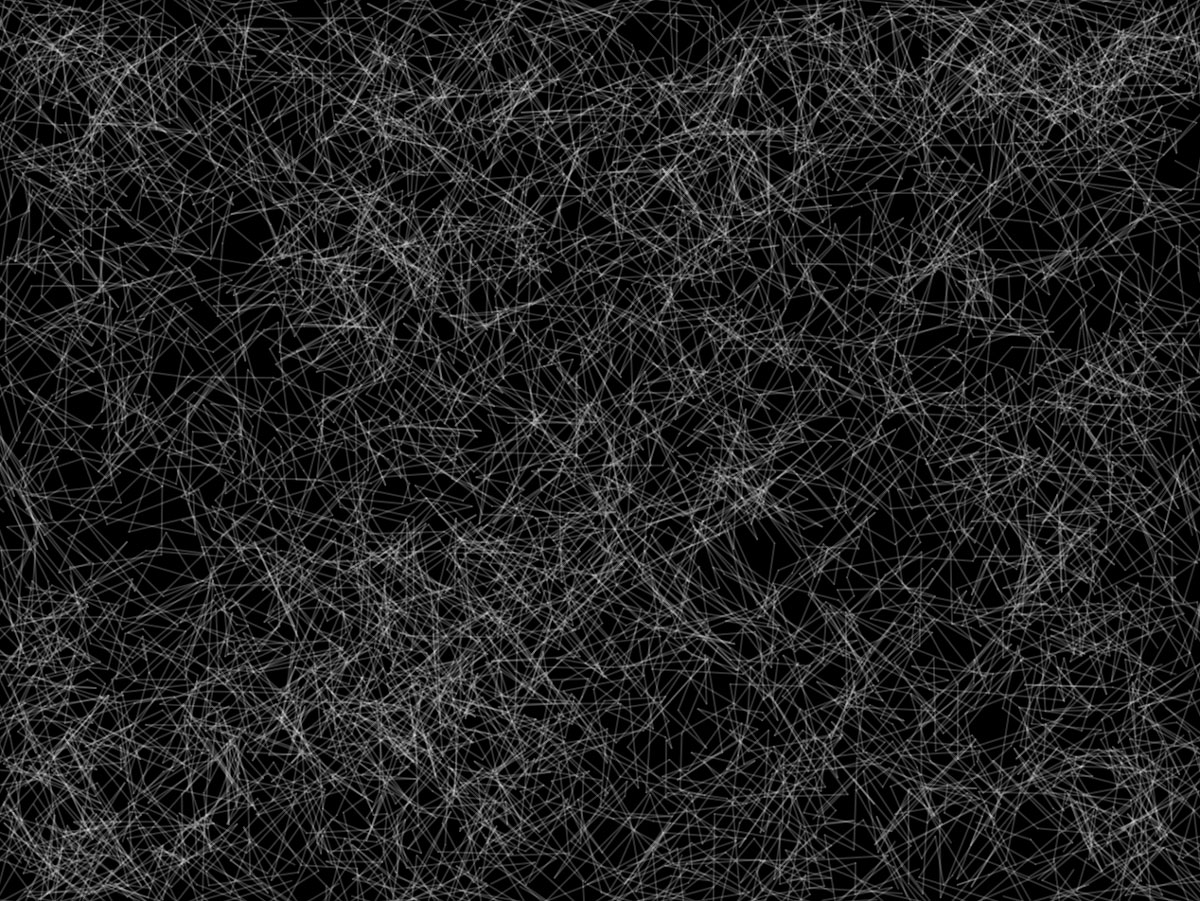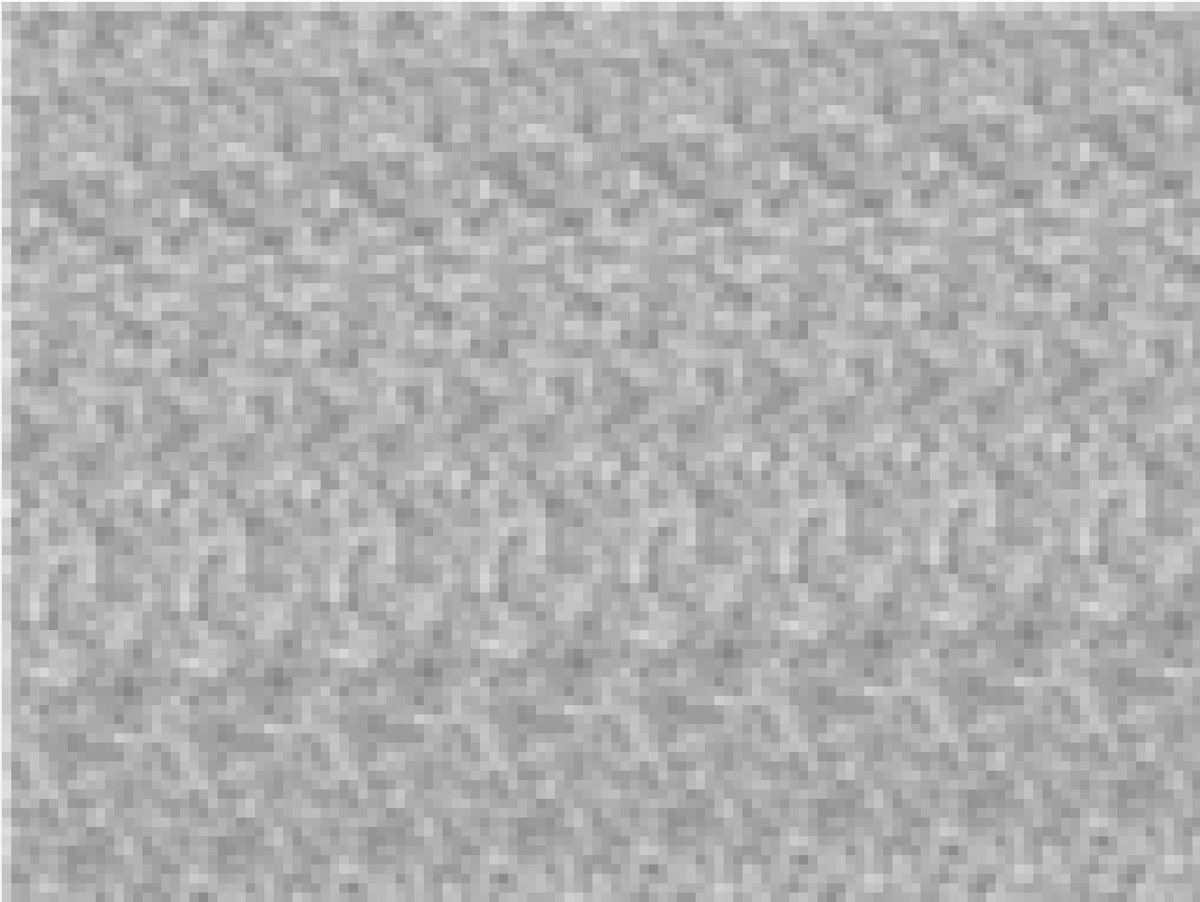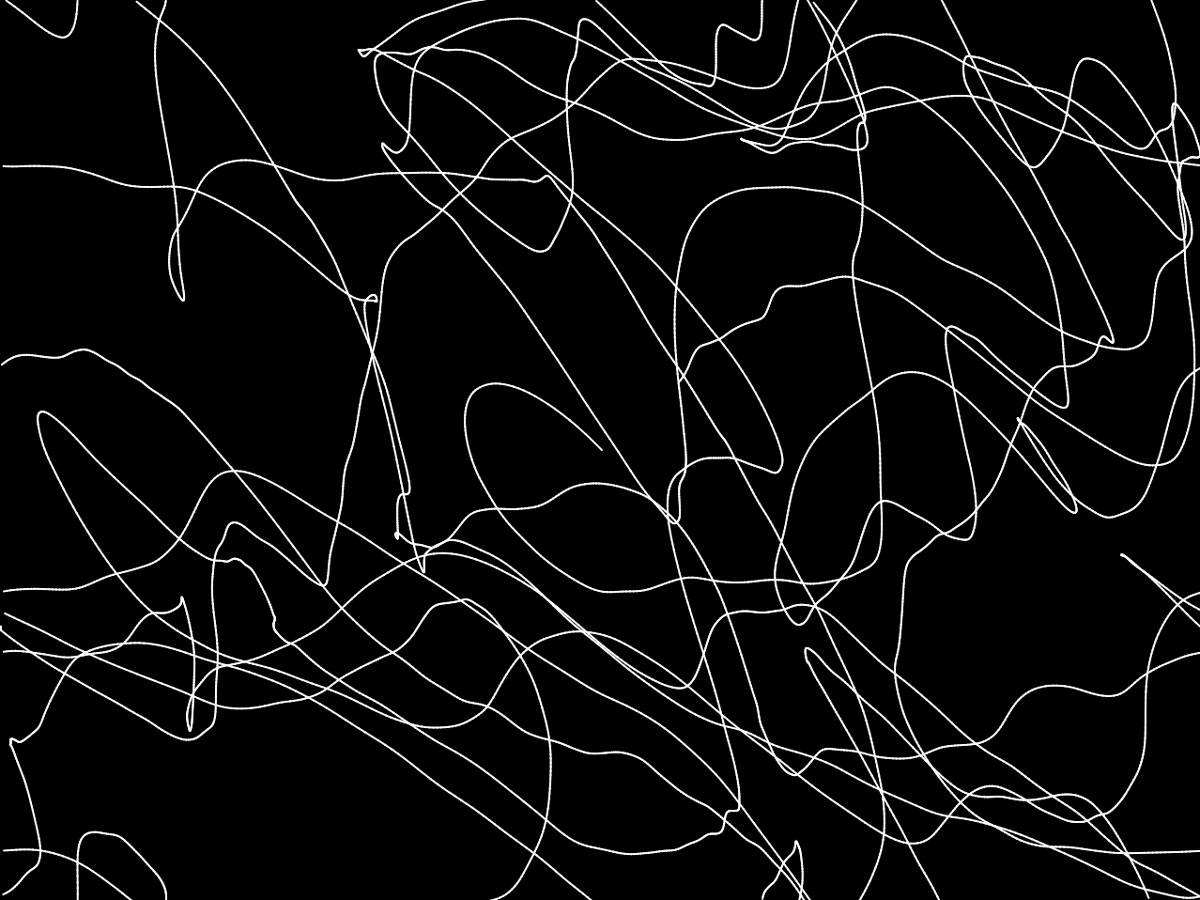### Generative Random Art

In this workshop, we ask the fundamental question of what randomness is and what it looks like. By asking students to express what they think random is, and by looking at what can constitute as randomness in nature, the workshop attempts at defining random. We ask the question of whether complete randomness is useful in creating visuals and explore ways to tame the randomness to create a desired controlled randomized visuals in p5.js, a javascript library for creating visuals.

Students will approach the concept of random first with pen and paper, to reflect on what their idea of random is. Then they will examine whether their idea of random is truly random and compare it with p5's idea of randomness. We will also compare two functions that can be used to generate random values in p5.js: random() and noise(). We will attempt at gaining a deeper knowledge of these functions by applying different visuals using these functions and go further into controlling it with other functions such as modulus(%) and map().

This workshop is presented as a final project for Teaching as Art class at NYU ITP.

#### What is RANDOM?

We first begin by asking the question of what random is. What is random? Can you draw something that is random?Here is a video explaining what random is, and whether something is truly random. If you roll a dice, or flip a coin, is the result you get a *random* result?

If nothing is random, or at least, finding something that is truly random is hard, then how are we generating *random* numbers computationally?

For details about how Javascript generates the pseudo random numbers, check out this ECMA Script documentation on Javascript's Math.random() function and this Hackernoon article.

#### Using random() in p5

We will first start off simple. Here is what you get if you use a random number to generate the x position of the ellipse.

#### Graphing random()

What are the properties of this graph? What's the width and height of this graph? What is the mid horizontal point of this graph? What is the maximum value and the minimum value?

#### Random Walks

As explained in the videos above, there is a concept of a random walk, which draws continuous lines of randomly generated x and y coordinates. Using the parameters of random we've discovered, how would be "control" this random walk?

The random() function that we use is PSEUDO random. How do we generate SAME random numbers for everyone? Click on the below sketch and press any keys to progress the "random" walk.

#### What is NOISE?

What comes to your mind when you think of a noisy drawing / image? How would you draw it on a piece of paper? How is this different from the random drawing that you did previously?In this workshop, we are going to talk specifically about Perlin noise. For more details about Perlin noise, read this blog post.

We will start simple with this one too. Here is what the horizontal movement of an ellipse looks like using noise() function.

#### Graphing noise()

What are the properties of this graph? What's the width and height of this graph? What is the mid horizontal point of this graph? What is the maximum value and the minimum value?

#### Noise Walks

Here is an adaptation of the random walk that we saw using the noise() function. What are the differences? What are the similarities?

#### Random vs Noise

Now lets look at how we can create different emotions to generate lines and shapes using random() and noise().

Below are two simple drawing tools, using random() and noise().

This is how we could animate a circular edged shape, to create a blob like movement, using random() and noise().

#### Code Example: Starry Ocean

Using the different characteristics of random() and noise(), this is an example of how the two different functions could be used to create a complete generated scene.

#### References:

Here are some references and inspirations for the content of this workshop.

Choreographic Interventions, Mimi Yin

Chapter 3: The Wrong Way to Draw A Line, Generative Art, Matt Pearson

Random Walks, Nature of Code, Daniel Shiffman

The random() Function, Coding Train, Daniel Shiffman

Perlin Noise, Coding Train, Daniel Shiffman